Skip to content
Related Articles
Two Poles and Cable Puzzle
• Difficulty Level : Medium
• Last Updated : 17 Mar, 2021

Problem Statement :
There are two poles of equal height – 15 meters. One cable with a length of 16 meters is hanging between those two poles. If the height from the center of the cable to the earth is 7 meters then what is the distance between the two poles?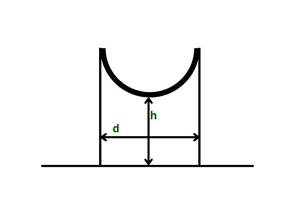In mathematical notations, we have ‘h’ representing the height from the center of the cable to earth, and we have ‘d’ as the distance between two poles. At what value of d, the value of h will be 7 meters?

Solution :
Consider the initial position of the cable to be straight. The length of the cable is 16 meters hence the distance between poles is 16 meters and the height from the center of the cable to the earth is 15 meters.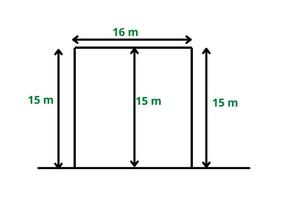So we can have the two parameters d = 16 meters and h = 15 meters. Now, if you bring the two poles closer to each other than the cable starts becoming a parabola, and we think that the cable is x meters lower than its previous position.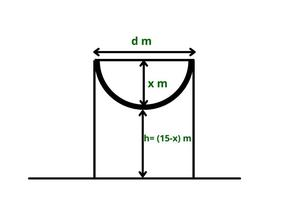Now think of calculating the value of x. Do you need to use the parabola equation?

The answer is NO. We need not use any parabola equation. Just keep on analyzing the different distances between the two poles. So if you bring the two poles further closer to each other than the center of the cable is much lower than its initial position and if we bring the two poles very close to each other such that the distance between them is 0 meters. Then we see that the cable is exactly divided into two halves.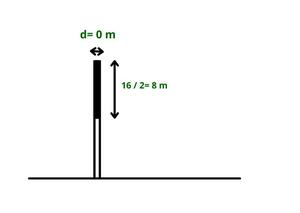When the length of the cable is 16 meters then the apparent length is 16/2 i.e. 8 meters and the height is (15-8) = 7 meters.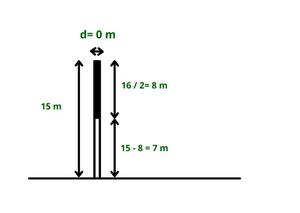And the puzzle was to find the value of d for which value of h = 7 meters. So the required value of d is 0 meters.

Therefore, if the height from the center of the cable to the earth is 7 meters then the distance between that two poles is 0 meters.

My Personal Notes arrow_drop_up